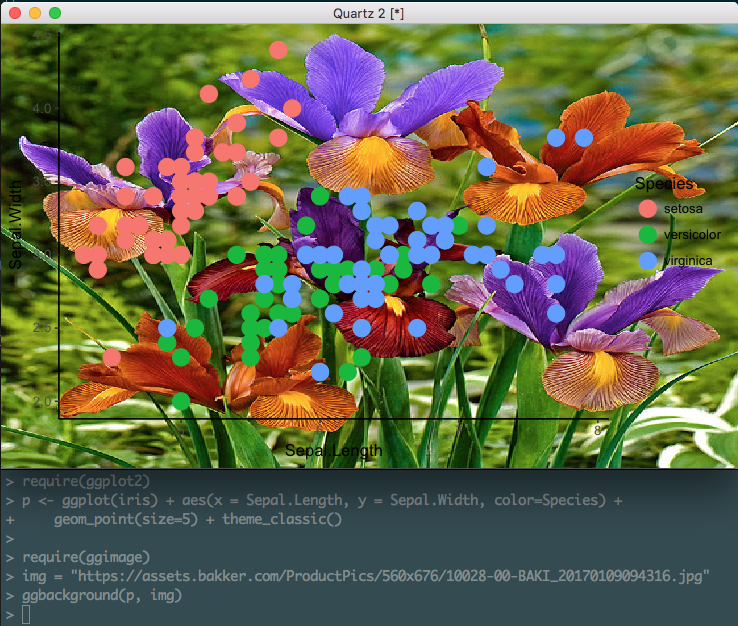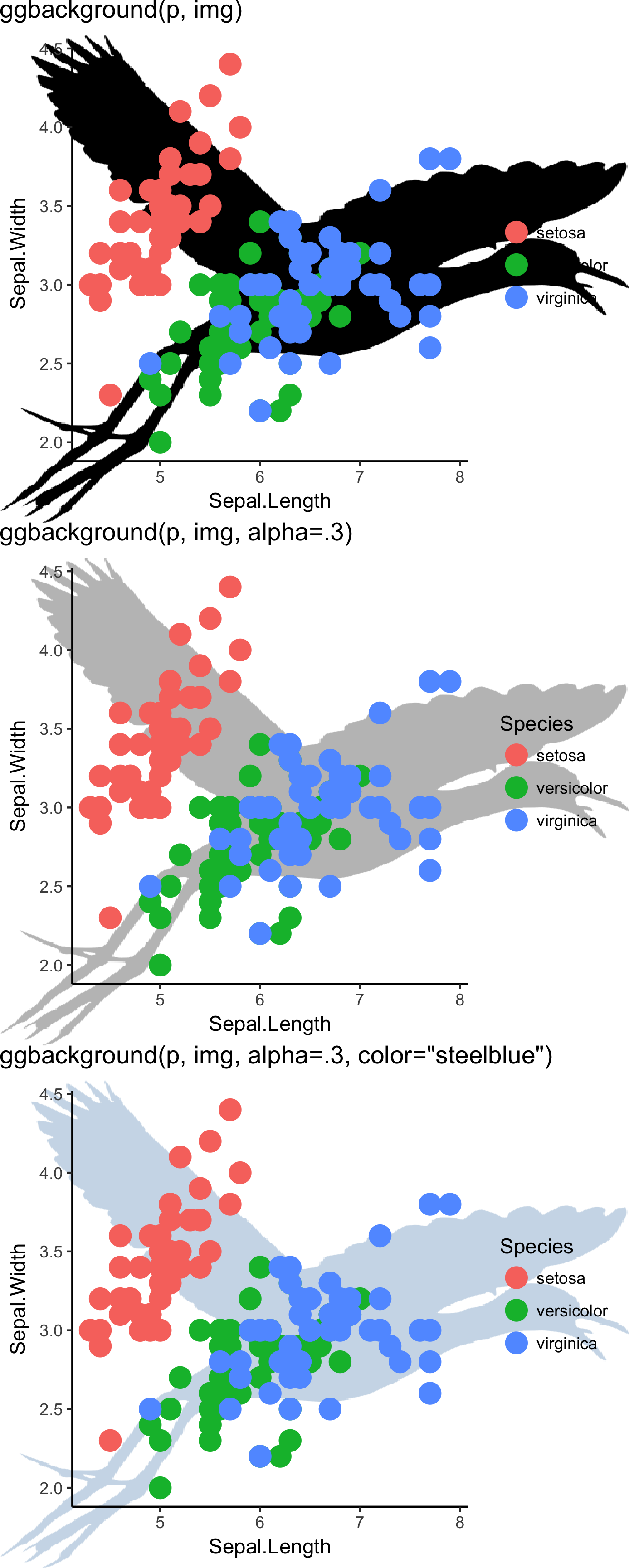ggimage 0.1.4 is available on CRAN.

This release introduces a new function called ggbackground for setting image background as ggplot canvas.

require(magick)
require(ggplot2)
require(ggimage)
require(ggplot2)
p <- ggplot(iris) + aes(x = Sepal.Length, y = Sepal.Width, color=Species) +
geom_point(size=5) + theme_classic()

Suppose we have the above ggplot object, p, the only thing we need to do is passing the p with an image file name (local or remote) to ggbackground, as demonstrated below:

require(ggimage)
img = "https://assets.bakker.com/ProductPics/560x676/10028-00-BAKI_20170109094316.jpg"
ggbackground(p, img)

This image file is too colorful and it is hard to see the patterns of the data points. We can manipulate the image using the functions provided by magick package. For example, the following function emulate a classic high-pass filter from photoshop:

require(magick)
ggbackground(p, img,
image_fun = function(x) image_negate(image_convolve(x, 'DoG:0,0,2')))Here are examples of setting transparency and color:

img = "http://phylopic.org/assets/images/submissions/bf5fe2c5-1247-4ed9-93e2-d5af255ec462.512.png"
p1 = ggbackground(p, img) + ggtitle("ggbackground(p, img)")
p2 = ggbackground(p, img, alpha=.3) + ggtitle("ggbackground(p, img, alpha=.3)")
p3 = ggbackground(p, img, alpha=.3, color="steelblue") + ggtitle('ggbackground(p, img, alpha=.3, color="steelblue")')
cowplot::plot_grid(p1, p2, p3, ncol=3)